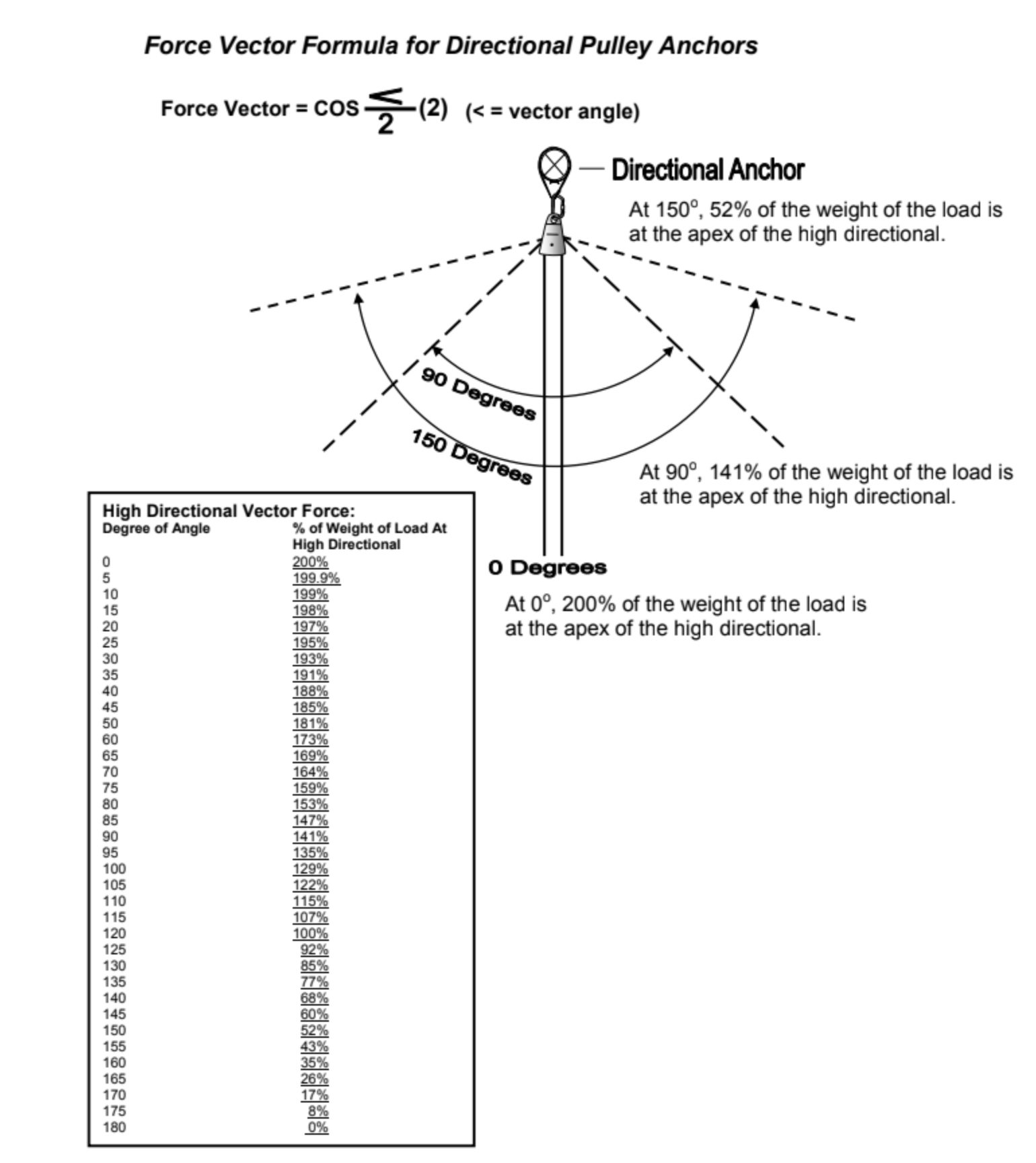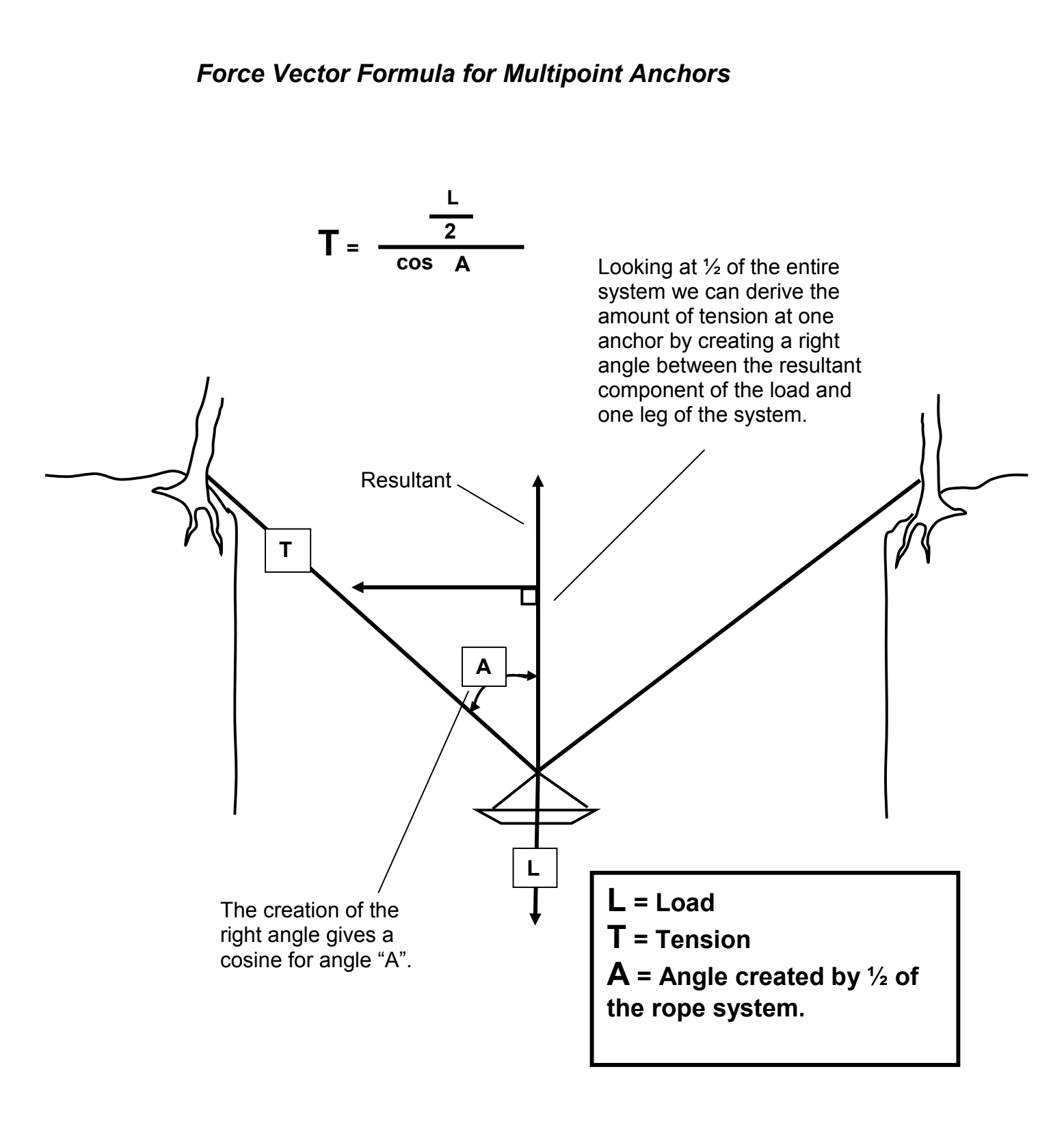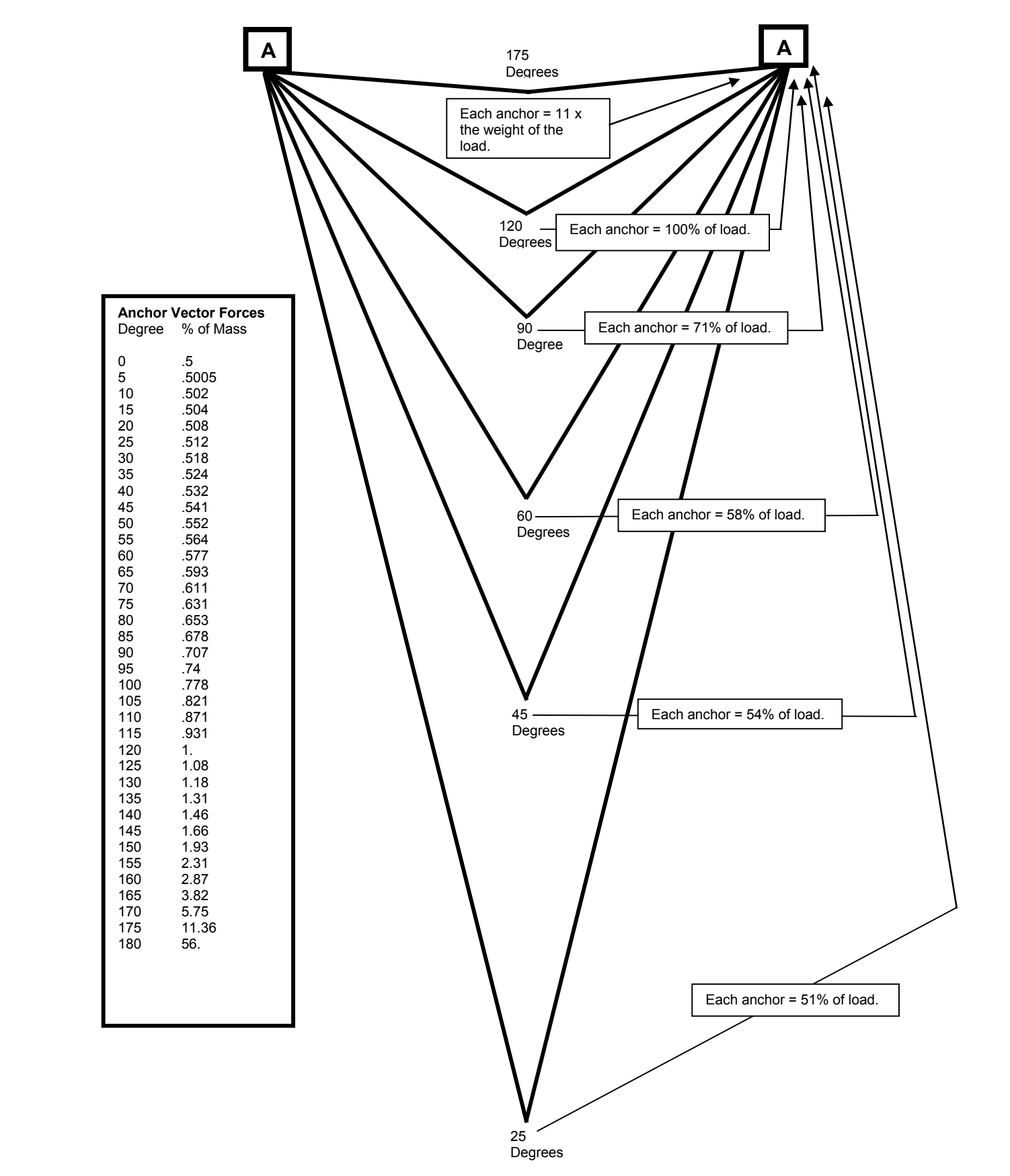## Written By: Lance Piatt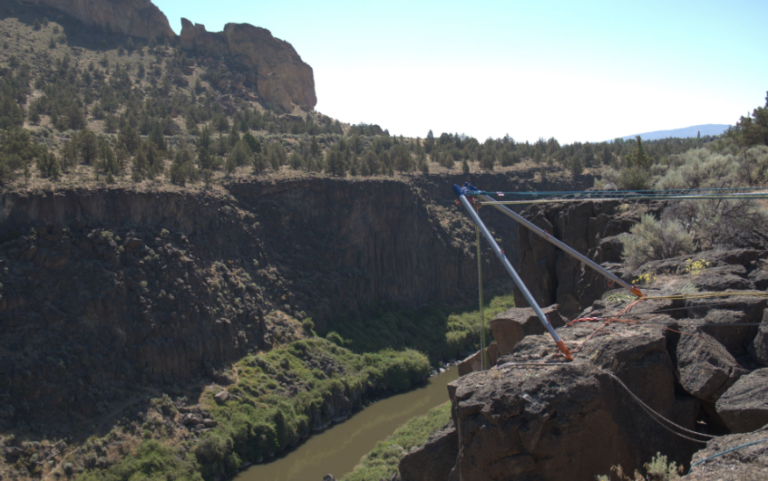Force multipliers and the four fundamentals of mechanics are important to understand in order to be a successful creator. The force multiplier is the means by which you can increase the force that you output, while the four fundamentals of mechanics are what keep your creation stable and functioning. If you want to be a successful creator, it is important to understand these concepts and apply them in your work.

This is a pretty hard book to open for many.  The only real way to get it, is to “get into it”.

We’ll start with some opening credits and some generalities, then jump into the deeper end of the pond.  It should be understanding… this is a very deep pond!

So what is or are Force Multipliers?

First off, “force multiplication” is a great term… consider it a military term;  Strategic and Tactical in nature.

“A factor, such as better positioning or equipment, which increases a unit’s combat potential, allowing it to fight on a par with a larger force.”  An example of this would be using a hammer; it multiplies the magnitude of the force you’re exerting and concentrates that force into a small area, making it easy to drive a nail in a single stroke. Saws, screwdrivers, and other tools work the same way — they Amplify and concentrate a small input into a larger output.

Force multipliers are a person or thing that dramatically increase the effectiveness of an item or group. There are four fundamentals of mechanics that can help create powerful force multipliers.

We see force multipliers everywhere, everyday and all the time.

A force multiplier is any factor or condition that contributes to the over all stress of a rope system during a dynamic state. Rating this dynamic state or rope movement/stress is best represented as the Dynamic System Safety Factor (DSSF). In other words, what is our weakest link during the operation of our rope system at its point of greatest stress, typically during onset of a hauling process?

For the specifics of rescue rigging

What are the 4 Fundamentals of Mechanics? Three are absolutes and one is a derivative of the other three.

1. Mass
2. Length
3. Time
4. Force

Force is not an absolute, it can be multiplied through various means. Force has two components to it… and these two characteristics create a “vector”.

What is a Vector?

• Direction
• Magnitude

Force Vectors
To understand rigging is to understand at least some aspects of trigonometry and vector physics. The knowledge of angles, components, and resultants is synonymous to quality rope rigging. To study vectors is to study the physical qualities of force that has both direction and magnitude.

Let’s do a recap on this subject from Volume One of Urban Technical Rescue, and then expand our study from there.

A force vector may be graphed or represented as a simple arrow, also more commonly referred to in math as a component. This component will always indicate the direction of the force.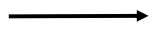When we compare the length of one force vector component to the length of another force vector component, we not only have the direction of each vector, but we now can compare their magnitude relative to each other.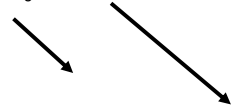Therefore, the component that is the longest has a greater magnitude. In fact, simply by comparing the two components we could deduct that the second is about 2 times more powerful or stronger than the first.

When two components come together we have now created an angle. The resultant is the force vector that is created by the joining of these two components.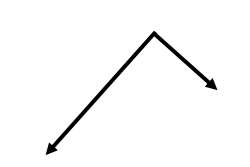To determine the resultant draw a parallelogram from the given angle. Than draw a new line (component) from the original angle (resultant) to its opposite angle of the parallelogram. By comparing the length of the resultant component to the components of the angle, we can easily see which component of this system is producing the greatest force simply by determining, which is the longest.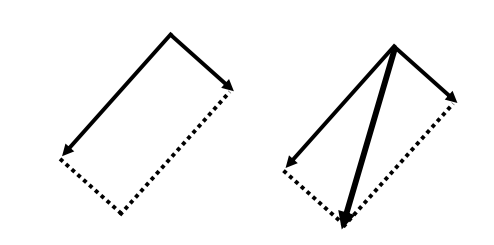When components of rope go through a pulley, the pulley equalizes the components of rope and renders them equal. The resultant (in this case, the pulley) will always seek the location halfway between the two rope components.

The center of any tensioned pulley will always point to the exact location and direction of the resultant force at work on the associated anchor.

Again, the resultant of any pulley will find the middle point between the legs of rope going in and coming out of the pulley. The use of the parallelogram still works well in determining the resultant force, its direction and magnitude.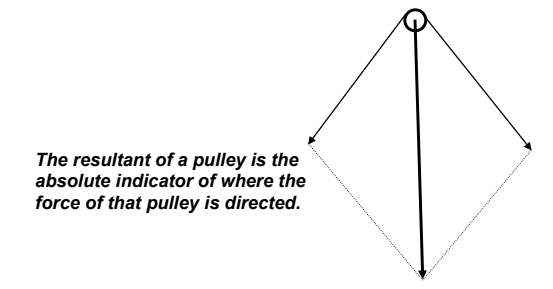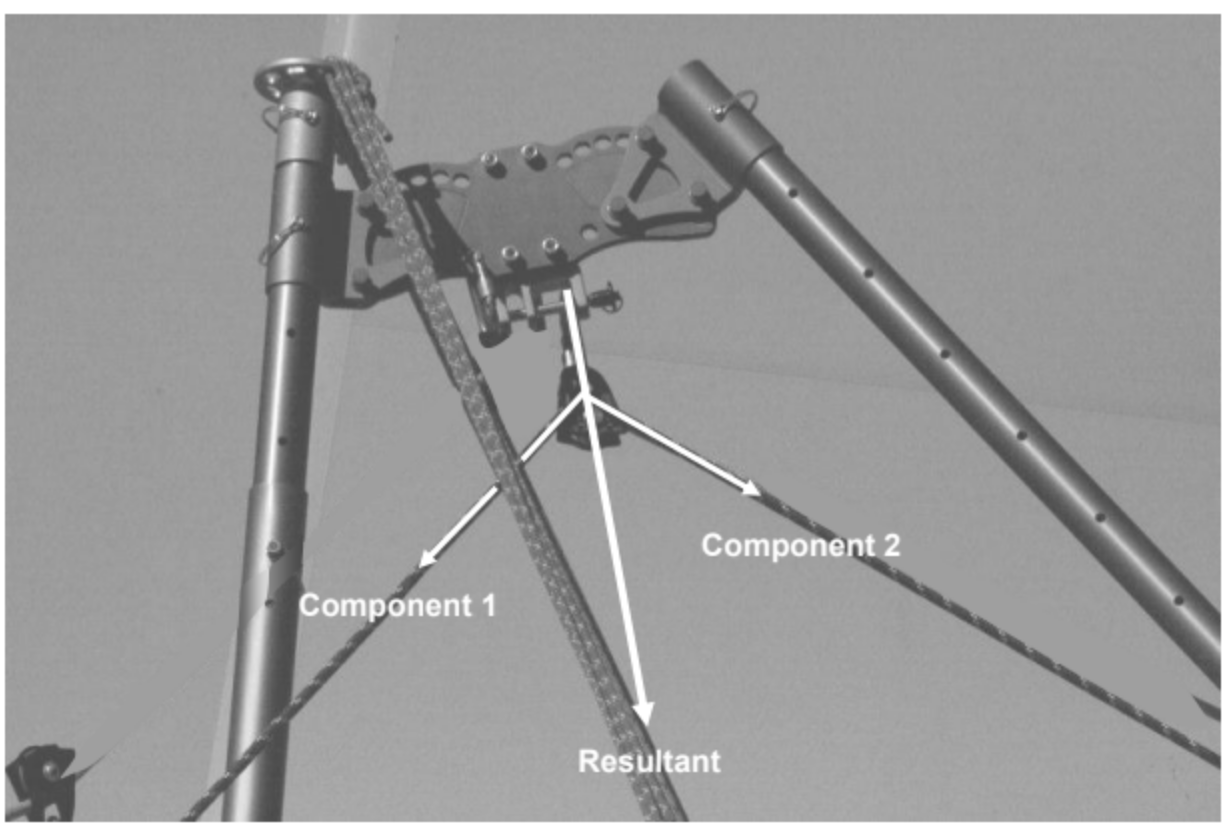One of the most misunderstood issues of rope rigging is the analysis of the angle of a multipoint anchor system versus the same angle of a directional pulley. Most rope rescue personnel have committed to rote memory that with a 90o angle, each anchor of a 2 by1 multipoint anchor system will receive 71% of the load, or with a 150o angle each anchor will receive 200% of the load. Given the same angles of rope going in and coming out of a directional pulley, when asked to give the force on the directional pulley anchor, many rescuers will erroneously

Study the two right angle rope systems below; both have two anchors and both support a 100kg load. What percentage of the load is each anchor receiving?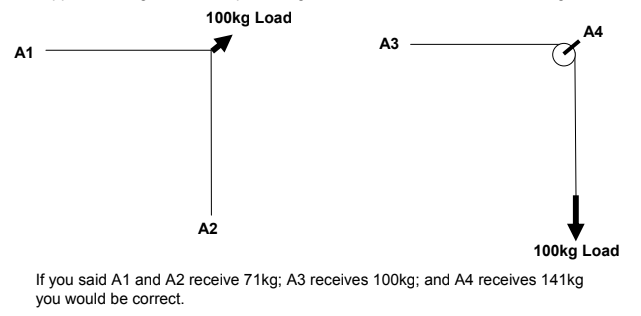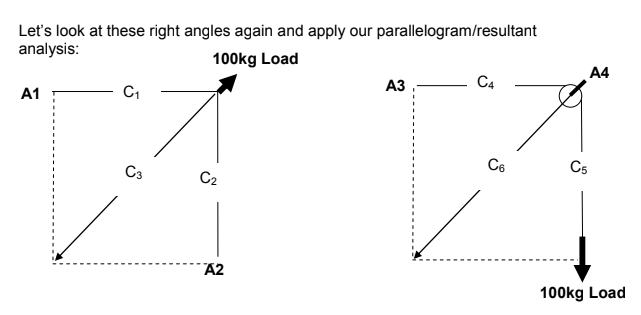The 100kg load is our known value, whatever component the load is connected to represents 100; the remaining two components may now be assigned a value simply by comparing their length. C3 = 100, therefore C1 and C2 are 71% of C3. C5 = 100, therefore C4 = 100% of C5 and C6 = 141% of C5.

Although using parallelograms as mentioned above to determine the resultant of any given angle is a good rule of thumb method, ultimately, for field application, we must commit to rote memory the recognition of angles as they apply to either, 1) multipoint anchor systems or 2) directional pulley anchors.